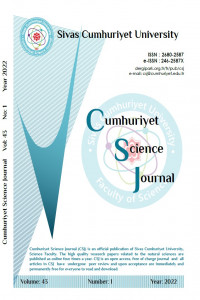Araştırma Makalesi

### Orthogonal Semiderivations and Symmetric Bi-semiderivations in Semiprime Rings

Yıl 2022, Cilt 43, Sayı 1, 105 - 112, 30.03.2022

### Öz

In this paper, orthogonality for symmetric bi-semiderivations is defined and some results are obtained when two symmetric bi-semiderivations are orthogonal. Also, this paper gives the notion of orthogonality between semiderivations and symmetric bi-semiderivations of a 2-torsion free semiprime ring and offers some results of orthogonality.In this paper, orthogonality for symmetric bi-semiderivations is defined and some results are obtained when two symmetric bi-semiderivations are orthogonal. Also, this paper gives the notion of orthogonality between semiderivations and symmetric bi-semiderivations of a 2-torsion free semiprime ring and offers some results of orthogonality.

### Kaynakça

•  Posner E. C., Derivations in prime rings, Proc. Amer. Math. Soc., 8 (1957) 1093-1100.
•  Maksa Gy., A remark on symmetric biadditive functions having nonnegative diagonalization, Glasnik. Mat., 15(35) (1980) 279-282.
•  Vukman J., Symmetric biderivations on prime and semiprime rings, Aequa. Math. 38 (1989) 245-254.
•  Vukman J., Two results concerning symmetric biderivations on prime rings, Aequa. Math., 40 (1990) 181-189.
•  Bergen J., Derivations in prime rings, Canadian Math. Bull., 26(3) (1983) 267-270.
•  Chang J. C., On semiderivations of prime rings, Chinese Journal Mathematics, 12(4) (1984) 255-262.
•  Bresar M., Vukman J., Orthogonal derivation and extension of a theorem of Posner, Rad. Mat. 5(2) (1989) 237-246.
•  Reddy C. J. S., Reddy B. R., Orthogonal symmetric bi-derivations in semiprime rings, International Journal of Mathematics and Statistics Studies, 4(1) (2016) 22-29.
•  Yılmaz D., Yazarlı H., (to appear), On symmetric bi-semiderivations of prime rings.
Yıl 2022, Cilt 43, Sayı 1, 105 - 112, 30.03.2022

### Kaynakça

•  Posner E. C., Derivations in prime rings, Proc. Amer. Math. Soc., 8 (1957) 1093-1100.
•  Maksa Gy., A remark on symmetric biadditive functions having nonnegative diagonalization, Glasnik. Mat., 15(35) (1980) 279-282.
•  Vukman J., Symmetric biderivations on prime and semiprime rings, Aequa. Math. 38 (1989) 245-254.
•  Vukman J., Two results concerning symmetric biderivations on prime rings, Aequa. Math., 40 (1990) 181-189.
•  Bergen J., Derivations in prime rings, Canadian Math. Bull., 26(3) (1983) 267-270.
•  Chang J. C., On semiderivations of prime rings, Chinese Journal Mathematics, 12(4) (1984) 255-262.
•  Bresar M., Vukman J., Orthogonal derivation and extension of a theorem of Posner, Rad. Mat. 5(2) (1989) 237-246.
•  Reddy C. J. S., Reddy B. R., Orthogonal symmetric bi-derivations in semiprime rings, International Journal of Mathematics and Statistics Studies, 4(1) (2016) 22-29.
•  Yılmaz D., Yazarlı H., (to appear), On symmetric bi-semiderivations of prime rings.

### Ayrıntılar

Birincil Dil İngilizce Matematik Natural Sciences Damla YILMAZ (Sorumlu Yazar) Erzurum Teknik Üniversitesi 0000-0002-6741-8669 Türkiye 30 Mart 2022 15 Ekim 2020 5 Mart 2022 Yıl 2022, Cilt 43, Sayı 1

### Kaynak Göster

 APA Yılmaz, D. (2022). Orthogonal Semiderivations and Symmetric Bi-semiderivations in Semiprime Rings . Cumhuriyet Science Journal , 43 (1) , 105-112 . DOI: 10.17776/csj.811057• Makale Dosyaları